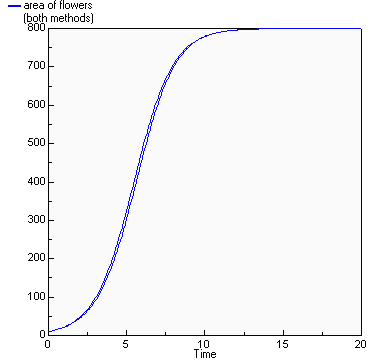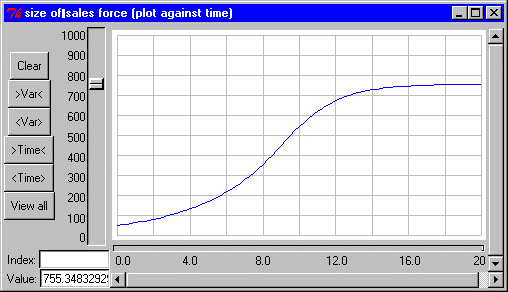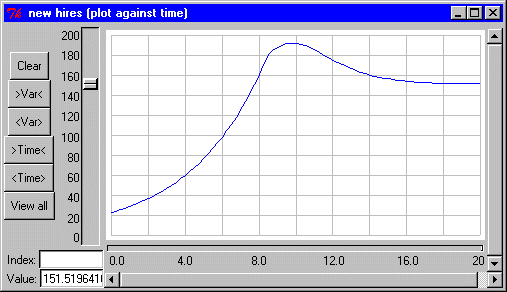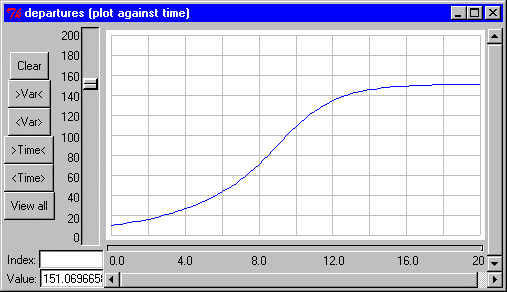# Lumped model

## Area covered in "flowers" compared with logistic growth model

ModelId:
flowers2
SimileVersion:
3.1+

This models the spatial spread of vegetation over an area. Here, the vegetation is taken to be �flowers�. This is not a spatial model, in the usual sense where space is divided up into discrete units. Rather, the area covered by the flowers is represented by a single state variable, with an inflow representing increase in area (spread), and an outflow representing decrease in area (death of flowers).

Equations:

Compartments:

area of flowers
Initial value = 10
Rate of change = + growth + decay
Comments: hectares (OK, Ford says acres, but we are in the 21st century now).

Flows:
decay = area_of_flowers*decay_rate

growth = area_of_flowers*actual_growth_rate

Variables:
A of t = Azero*exp(r*time(1))/(1+Azero*(exp(r*time(1))-1)/K)

actual growth rate = intrinsic_growth_rate*growth_rate_multiplier
Comments: dimensionless

area of flowers (both methods) = [area_of_flowers,A_of_t]

fraction occupied = area_of_flowers/suitable_area

growth rate multiplier = graph(fraction_occupied)

intrinsic growth rate = 1
Comments: I.e. 100% per year

Parameters:
Azero = 10
Comments: Initial area of flowers (hectare)

decay rate = 0.2
Comments: Proportion decaying (per year)

K = 800
Comments: "Maximum value that the flowered area would reach over time"

r = intrinsic_growth_rate-decay_rate

suitable area = 1000
Comments: hectares (see 'area of flowers'...)

Results:References:

Ford, A (1999) Modeling the Environment.

ModelId:
cogrowth1
SimileVersion:
5.x

## Description

This models the changing size of the sales force of a company, using feedbacks between the size of the sales force, the success of this sales force in selling goods, and further recruitment.

Source: Andrew Ford (1999): Modelling the Environment, p.64Equations:

Compartments:

size of sales force
Initial value = 50
Rate of change = + new_hires - departures

Flows:
new hires = hiring_fraction*(budgeted_size_of_sales_force-size_of_sales_force)
departures = size_of_sales_force*exit_rate

Variables:
annual revenue in millions = widget_sales*widget_price/1000000
budgeted size of sales force = sales_dept_budget*1000000/average_salary
effectiveness in widgets per day = graph(size_of_sales_force)
sales dept budget = fraction_to_sales*annual_revenue_in_millions
widget sales = size_of_sales_force*effectiveness_in_widgets_per_day*365

Parameters:
average salary = 25000
exit rate = 0.2
fraction to sales = 0.5
hiring fraction = 1
widget price = 100

Results:Model tags: